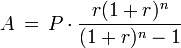# Everyday Calculation

Free calculators and unit converters for general and everyday use.

Calculators » Finance » Loan EMI

## Loan EMI Calculator

Our online tools will provide quick answers to your calculation and conversion needs. On this page, you can calculate equated monthly installments (EMI) and total interest payable on bank loans including personal loan, home loan, car loan among others.

Result window

Download: Use this EMI calculator offline with our all-in-one calculator app for Android and iOS.

To calculate loan repayment where payment is made in daily, quarterly, half-yearly or yearly installments, use this advanced loan calculator.

#### EMI calculation

The EMI formula (more commonly known as Amortization):Where,
P = principal amount borrowed,
A = monthly payment or emi,
r = interest rate in % divided by 12,
n = total number of months